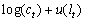John Hassler

Kjetil Storesletten

Åsa Johansson

March 21, 1997

EXAM
Macroeconomics I
5 points

As usual the exam consists of three parts, I Short Questions, II Problem and III Essay. Choose 5 questions from part I, one from part II and one from part III. A pass will require 60 points (out of 100) and a pass with distinction 80 points. Make sure you spend your time wisely. One of us will come down after 90 minutes, it is advisable that you read through the exam before that.I Short answers

Choose 5 out of the following 6. 1 page per question should be more than enough for your answers. Worth 10 points each.

1. Evaluate the following statement: "A temporarily high government spending, like a war, should be financed by temporarily high taxes."
2. Evaluate the following statement: "Humans do not live for ever. The probability of dying implies that the Ricardian Equivalence theorem cannot hold."
3. Is the following statement true, false or uncertain? "If an individual’s savings behavior is unaffected by risk one knows he is risk-neutral."
4. In a standard Solow model, let Y=Ka N1-a with a =0.5. Let population growth rate be 0.02, the savings rate = 0.10 and depreciation 0.10. Compute the steady state consumption per capita.
5. The standard Ramsey model predicts convergence; production and consumption growth is higher in countries with low initial capital stocks. What would happen with that prediction if we considered a small open economy, with free flows of capital?
6. Is the following statement true, false or uncertain? If the agent’s subjective discount rate is positive, then the steady state capital stock in a Ramsey model is always lower than the Golden Rule capital stock.

II Problem

Do one of the following two problems (choose 8 or 9). Provide a strict formal argument. Worth 25 points

7. Taxation in the Ramsey model

Consider n representative individual who solves1

where U is a CARA type utility function, f is a standard concave production function, K is the capital stock, c is consumption and T is a tax.

1. Set up the current value Hamiltonian with its necessary conditions for optimality.
2. Express the optimality conditions and the transition equation for K as a system of two differential equations in K and c. (Hint; Take time derivatives of the condition for the maximum of the Hamiltonian). Present your results as a phase diagram in K and C. Make sure you indicate movements in the phase diagram in all areas and any potential saddle path.
3. What are the effects of an unexpected permanent increase in the tax rate. Indicate transitions and the long run effect in the phase diagram. Describe in words what is happening and provide intuitive economic explanations.
4. Now consider an unexpected temporary increase in the tax rate, between, say, time t and T. Indicate transitions and the long run effect in the phase diagram. Describe in words what is happening and provide intuitive economic explanations.
5. Lastly, assume that at t it is announced that at T the tax rate will permanently be raised at T >t. Describe in words what is happening and provide intuitive economic explanations.

8. Intertemporal substitution of labor

Assume that

• The agents have preferences over leisure lt and consumption ct and maximizewhere u(.) is concave and increasing.
• Markets are complete.
• Labor input is indivisible, i.e. one can work h0 hours or not at all (so leisure is 1- h0 or 1, say).
1. Show that aggregation holds in this economy in the sense that, by introducing a particular set of assets (lotteries), the aggregate outcomes could have been generated by a representative consumer (with preferences not necessarily the same as those of the underlying agents, see (b) below).
2. Show that the preferences of the representative agent are linear in aggregate labor input, regardless of the concavity of u(.).
3. What does the findings in (b) teach us about the willingness to substitute leisure across time for the representative agent versus the individuals in the economy? Explain why this can to some extent resolve the criticism levied against RBC theory.

III Essay

Choose 1 out of the following 2. Emphasize discussion rather than formalism. Worth 25 points.

9. Asset Pricing Models

The standard and consumption based CAPM are two variants of assets pricing models? How do they differ in their assumptions and implications? Describe some empirical tests of the two models and discuss their relative performance. Provide some hypothetical explanations for the empirical findings.